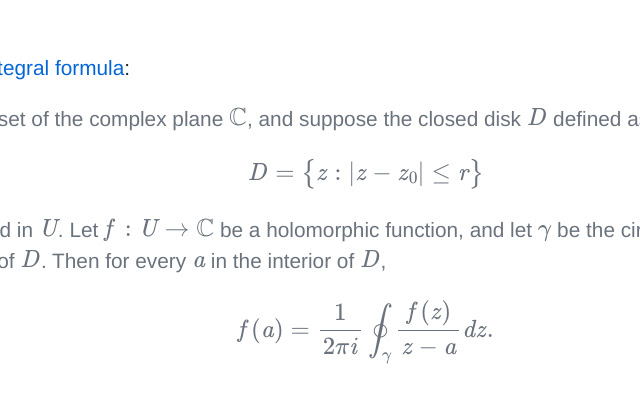# Green Pi

CRX id

## ingbbliecffofmmokknelnijicfcgolbDescription from extension meta

## Beautiful math wherever you want.

Image from storeDescription from store Green Pi is a browser extension that renders LaTeX-style mathematics on pages that don't otherwise render math, like GitHub. As an author, you simple need to include the URL https://github.com/nschloe/green-pi?activate&inlineMath=$somewhere in your page (e.g., your README.md). See https://github.com/nschloe/green-pi for all options. Then, the following text will be rendered with math. Let$U$be an open subset of the complex plane$\mathbb{C}$, and suppose the closed disk$D$defined as $$D = \bigl\\{z:|z-z_{0}|\leq r\bigr\\}$$ is completely contained in$U$. Let$f: U\to\mathbb{C}$be a holomorphic function, and let$\gamma$be the circle, oriented counterclockwise, forming the boundary of$D$. Then for every$a$in the interior of$D\$, $$f(a) = \frac{1}{2\pi i} \oint _{\gamma}\frac{f(z)}{z-a} dz.$$

### Statistics

Installs
49 history
Category
Rating
0.0 (0 votes)
Last update / version
2021-03-22 / 0.1.6
Listing languages